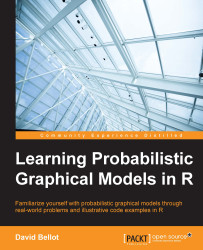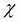•#### Learning Probabilistic Graphical Models in R#### Overview of this book

Probabilistic graphical models (PGM, also known as graphical models) are a marriage between probability theory and graph theory. Generally, PGMs use a graph-based representation. Two branches of graphical representations of distributions are commonly used, namely Bayesian networks and Markov networks. R has many packages to implement graphical models. We’ll start by showing you how to transform a classical statistical model into a modern PGM and then look at how to do exact inference in graphical models. Proceeding, we’ll introduce you to many modern R packages that will help you to perform inference on the models. We will then run a Bayesian linear regression and you’ll see the advantage of going probabilistic when you want to do prediction. Next, you’ll master using R packages and implementing its techniques. Finally, you’ll be presented with machine learning applications that have a direct impact in many fields. Here, we’ll cover clustering and the discovery of hidden information in big data, as well as two important methods, PCA and ICA, to reduce the size of big problems.
Learning Probabilistic Graphical Models in RCreditswww.PacktPub.comPrefaceFree Chapter
Probabilistic ReasoningExact InferenceLearning ParametersBayesian Modeling – Basic ModelsApproximate InferenceBayesian Modeling – Linear ModelsProbabilistic Mixture ModelsAppendixIndex## Variable elimination

The previous example is quite impressive and seems to be complex. In the following sections we are going to see how to deal with such complex problems and how to perform inference on them, whatever the model is. In practice, we will see that things are not as idyllic as they seem to be and there are a few restrictions. Moreover, as we saw in the first chapter, when one solves the problem of inference, one has to deal with the NP-hard problem, which leads to algorithms that have an exponential time complexity.

Nevertheless there are dynamic programming algorithms that can be used to achieve a high degree of efficiency in many problems of inference.

We recall that inference means the computing of a posterior distribution of a subset of variables, given observed values of another subset of the variables of the model. Solving this problem in general means we can choose any disjoint subsets.

Letbe the set of all the variables in the graphical model and let Y and E be two disjoint...